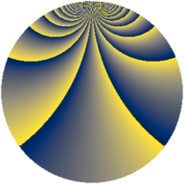# Properties

 Label 1840.2.baLevel $1840$ Weight $2$ Character orbit 1840.ba Rep. character $\chi_{1840}(47,\cdot)$ Character field $\Q(\zeta_{4})$ Dimension $132$ Sturm bound $576$

# Related objects

## Defining parameters

 Level: $$N$$ $$=$$ $$1840 = 2^{4} \cdot 5 \cdot 23$$ Weight: $$k$$ $$=$$ $$2$$ Character orbit: $$[\chi]$$ $$=$$ 1840.ba (of order $$4$$ and degree $$2$$) Character conductor: $$\operatorname{cond}(\chi)$$ $$=$$ $$20$$ Character field: $$\Q(i)$$ Sturm bound: $$576$$

## Dimensions

The following table gives the dimensions of various subspaces of $$M_{2}(1840, [\chi])$$.

Total New Old
Modular forms 600 132 468
Cusp forms 552 132 420
Eisenstein series 48 0 48

## Trace form

 $$132q + O(q^{10})$$ $$132q + 12q^{13} + 12q^{17} + 12q^{25} - 48q^{33} - 60q^{37} - 60q^{45} - 12q^{53} - 12q^{65} + 36q^{73} - 132q^{81} + 60q^{85} + 36q^{97} + O(q^{100})$$

## Decomposition of $$S_{2}^{\mathrm{new}}(1840, [\chi])$$ into newform subspaces

The newforms in this space have not yet been added to the LMFDB.

## Decomposition of $$S_{2}^{\mathrm{old}}(1840, [\chi])$$ into lower level spaces

$$S_{2}^{\mathrm{old}}(1840, [\chi]) \cong$$ $$S_{2}^{\mathrm{new}}(20, [\chi])$$$$^{\oplus 6}$$$$\oplus$$$$S_{2}^{\mathrm{new}}(80, [\chi])$$$$^{\oplus 2}$$$$\oplus$$$$S_{2}^{\mathrm{new}}(460, [\chi])$$$$^{\oplus 3}$$